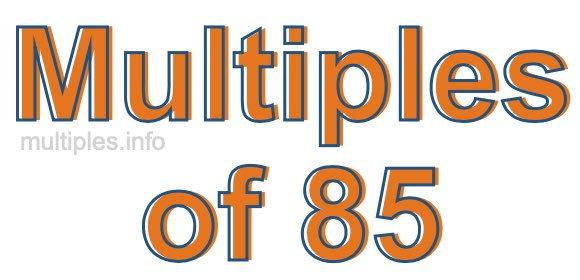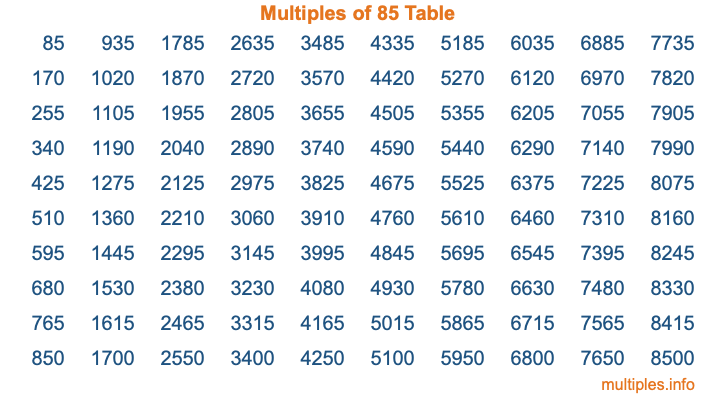Multiples of 85Welcome to the Multiples of 85 page. Here we will first teach you everything you will ever need to know about the multiples of 85, and then give you a study guide summary of everything we taught you to make sure you remember it all. Use this page to look up facts and learn information about the multiples of 85. This page will make you a multiples of eighty-five expert!

Definition of Multiples of 85
Multiples of 85 are all the numbers that when divided by 85 equal an integer. Each of the multiples of 85 are called a multiple. A multiple of 85 is created by multiplying 85 by an integer.

Therefore, to create a list of multiples of 85, you start with 1 multiplied by 85, then 2 multiplied by 85, then 3 multiplied by 85, and so on for as long as you want. Thus, the list of the first five multiples of 85 is 85, 170, 255, 340, and 425. To see a larger list of multiples of 85, see the printable image of Multiples of 85 further down on this page. We also have a category where you can choose any nth multiple of 85.

Multiples of 85 Checker
The Multiples of 85 Checker below checks to see if any number of your choice is a multiple of 85. In other words, it checks to see if there is any number (integer) that when multiplied by 85 will equal your number. To do that, we divide your number by 85. If the the quotient is an integer, then your number is a multiple of 85.

Is  a multiple of 85?

Least Common Multiple of 85 and ...
A Least Common Multiple (LCM) is the lowest multiple that two or more numbers have in common. This is also called the smallest common multiple or lowest common multiple and is useful to know when you are adding our subtracting fractions. Enter one or more numbers below (85 is already entered) to find the LCM.

Check out our LCM Calculator if you need more details about the Least Common Multiple or if you need the LCM for different numbers for adding and subtraction fractions.

nth Multiple of 85
As we stated above, 85 is the first multiple of 85, 170 is the second multiple of 85, 255 is the third multiple of 85, and so on. Enter a number below to find the nth multiple of 85.

th multiple of 85

Multiples of 85 vs Factors of 85
85 is a multiple of 85 and a factor of 85, but that is where the similarities end. All postive multiples of 85 are 85 or greater than 85. All positive factors of 85 are 85 or less than 85.

Below is the beginning list of multiples of 85 and the factors of 85 so you can compare:

Multiples of 85: 85, 170, 255, 340, 425, etc.

Factors of 85: 1, 5, 17, 85

As you can see, the multiples of 85 are all the numbers that you can divide by 85 to get a whole number. The factors of 85, on the other hand, are all the whole numbers that you can multiply by another whole number to get 85.

It's also interesting to note that if a number (x) is a factor of 85, then 85 will also be a multiple of that number (x).

Multiples of 85 vs Divisors of 85
The divisors of 85 are all the integers that 85 can be divided by evenly. Below is a list of the divisors of 85.

Divisors of 85: 1, 5, 17, 85

The interesting thing to note here is that if you take any multiple of 85 and divide it by a divisor of 85, you will see that the quotient is an integer.

Multiples of 85 Table
Below is an image of the first 100 multiples of 85 in a table. The table is in chronological order, column by column. The first column has the first ten multiples of 85, the second column has the next ten multiples of 85, and so on.The Multiples of 85 Table is also referred to as the 85 Times Table or Times Table of 85. You are welcome to print out our table for your studies.

Negative Multiples of 85
Although not often discussed or needed in math, it is worth mentioning that you can make a list of negative multiples of 85 by multiplying 85 by -1, then by -2, then by -3, and so on, to get the following list of negative multiples of 85:

-85, -170, -255, -340, -425, etc.

Multiples of 85 Summary
Below is a summary of important Multiples of 85 facts that we have discussed on this page. To retain the knowledge on this page, we recommend that you read through the summary and explain to yourself or a study partner why they hold true.

There are an infinite number of multiples of 85.

A multiple of 85 divided by 85 will equal a whole number.

85 divided by a factor of 85 equals a divisor of 85.

The nth multiple of 85 is n times 85.

The largest factor of 85 is equal to the first positive multiple of 85.

85 is a multiple of every factor of 85.

85 is a multiple of 85.

A multiple of 85 divided by a divisor of 85 equals an integer.

85 divided by a divisor of 85 equals a factor of 85.

Any integer times 85 will equal a multiple of 85.

Multiples of a Number
Here you can get the multiples of another number, all with the same attention to detail as we did for multiples of 85 on this page.

Multiples of
Multiples of 86
Did you find our page about multiples of eighty-five educational? Do you want more knowledge? Check out the multiples of the next number on our list!